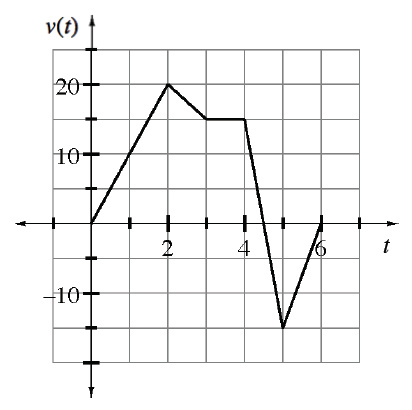### Home > APCALC > Chapter 3 > Lesson 3.1.1 > Problem3-16

3-16.

After class, Stevie travels in a straight hallway with a velocity shown in the graph at right, where $t$ is measured in minutes and $v(t)$ is measured in feet per minute.

1. Explain what is happening when $t > 4.5$ minutes.

Notice that the velocity is negative when $t > 4.5$.

When velocity is negative, Stevie moves back towards his starting position.

2. Calculate the total distance Stevie traveled.

Total distance is the area under a speed graph. Speed is the absolute value of velocity.

A speed graph is the absolute value of a velocity graph...
$\text{Area under a speed graph = total distance}$.
$\text{Area under a velocity graph = displacement}$.

3. If Stevie only travels in the straight hallway, how far does he end up from his original starting place?

Distance from starting point is known as 'displacement.'
Displacement is the area under a velocity graph.

4. What is Stevie’s acceleration at $t = 1$?

Acceleration is slope on a velocity graph.

5. When is Stevie’s acceleration equal to zero?

See hint in part (d).